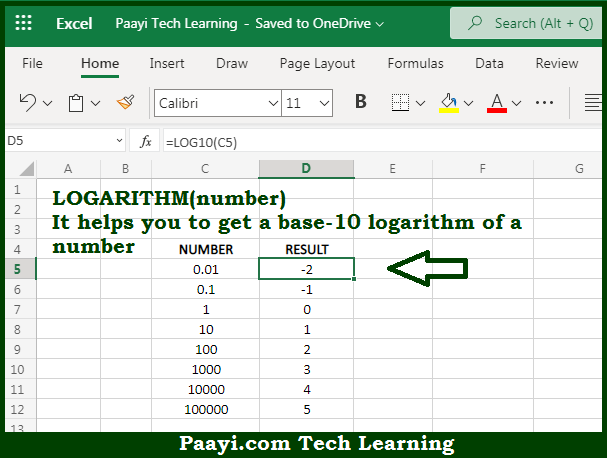# Learn How to Use Microsoft Excel LOG10 Function

Written by | 0 Comments | 545 Views

In this article, you will learn how to use the Microsoft Excel LOG10 function and its prime function in Microsoft Excel. You will also get to know the Microsoft Excel LOG10 function return value and syntax with the help of some examples.

Microsoft Excel LOG10 Function

The main function of the Microsoft Excel LOG10 function is to get the base 10 logarithm of the number. So, with the help of the LOG10 function, you can able to return the base 10 logarithm of a given number. It should be noted that the base value defaults to 10, in case it is not provided. So, with the help of the LOG10 function, you can able to return the base 10 logarithm of the given number.

Return Value of LOG10 Function

The return value will be the base 10 of the logarithm.

Syntax of LOG10 Function

=LOG10(number)

Where the arguments:

• number: This is the number of which you want to get the base 10 logarithm.

## How to Use Microsoft Excel LOG10 Function?So we know that Microsoft Excel LOG10 function you can able to return the base 10 logarithm of the given number. It should be noted that the base value defaults to 10, in case it is not provided. So, with the help of the LOG10 function, you can able to return the base 10 logarithm of the given number.